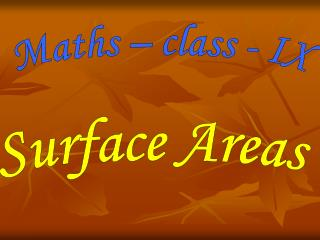# Maths – class - IX - PowerPoint PPT PresentationDownload PresentationMaths – class - IX

Maths – class - IX
Download Presentation## Maths – class - IX

- - - - - - - - - - - - - - - - - - - - - - - - - - - E N D - - - - - - - - - - - - - - - - - - - - - - - - - - -
##### Presentation Transcript

1. Maths – class - IX Surface Areas

2. Surface Areas Let us find out the surface areas of all these objects ! Cube Cuboid Cylinder Cone Sphere

3. Surface area of a cuboid • A bundle of many sheets of paper makes up a cuboid. • We have used six rectangular pieces to cover the complete outer surface of the cuboid. • Area of 6 rectangles = 2(lxb) + 2(bxh) + 2(lxh) • Surface area of a cuboid= 2 (lb + bh + hl)

4. Surface area of a cube • A cuboid, whose length, breadth and height are all equal, it is called a Cube. If each edge of the cube is a, then the surface area of this cube would be: 2(axa+axa+axa),i.e.,6a², giving us – Surface area of a cube =6a² where a is the edge of the cube.

5. Surface area of a right circular cylinder • Take a number of circular sheets of paper and stack them up, if the stack is kept vertically up, we get a Right circular cylinder, since it has been kept at right angles to the base, and the base is circular.

6. The area of the rectangular sheet gives us the curved surface area of the cylinder. The length of the sheet is equal to the circumference of the circular base which is equal to 2חr. So, curved surface of the cylinder = area of the rectangular sheet = length x breadth = perimeter of the base of the cylinder x h = 2 חr x h Therefore, Curved surface area of a cylinder = 2חrh.

7. If the top and the bottom of the cylinder are also to be covered, then we need two circles to do that, each of radius r, and thus having an area of חr² each, giving us the total surface area as 2 חrh + 2 חr² = 2 חr²(r+h) . So, Total surface area of a cylinder =2 חr (r +h) Where h is the height of the cylinder and r is it’s radius.

8. Surface area of a right circular cone • The area of each triangle = ½ x base of each triangle x l. So, area of the entire piece of paper = sum of the areas of all the triangles = ½ b1l+ ½b2l+ ½b3l+… = ½l(b1+b2+b3+…) = ½ x l x length of entire curved boundary of the fig 3 (as b1+b2+b3+… makes up the curved portion of the fig) But the curved portion of the figure makes up the perimeter of the base of the cone and the circumference of the base of the cone = 2 חr, where r is the base radius of the cone. So, Curved surface area of a cone = ½ x 1 x 2 חr = חrl. Where r is it’s base radius and l. it’s slant height. TSA of a cone = חr l. + חr² = חr (l+r)

9. Surface area of a sphere • The string which had completely covered the surface area of the sphere, has been used to completely fill the regions of four circles, all of the same radius as of the sphere. • This suggests that the surface area of a sphere of radius r = 4 times the area of a circle of radius r =4 x (חr²) So, Surface area of a sphere =4 חr². Where r is the radius of the sphere.

10. Evaluation • A cubic box has each edge 10 cm and another cuboidal box is 12.5 cm long, 10 cm wide and 8 cm high. Which box has the grater surface area and by how much? • A cylindrical pillar is 50 cm in diameter and 3.5 m in height. Find the cost of painting the curved surface of the pillar at the rate of Rs 12.50 per m² . • Diameter of the base of a ice cream cone is 10.5 cm and its slant height is 10 cm. Find its curved surface area. • Find the surface area of a sphere of radius: 14 cm. • Find the radius of a sphere whose surface area is 154 cm².